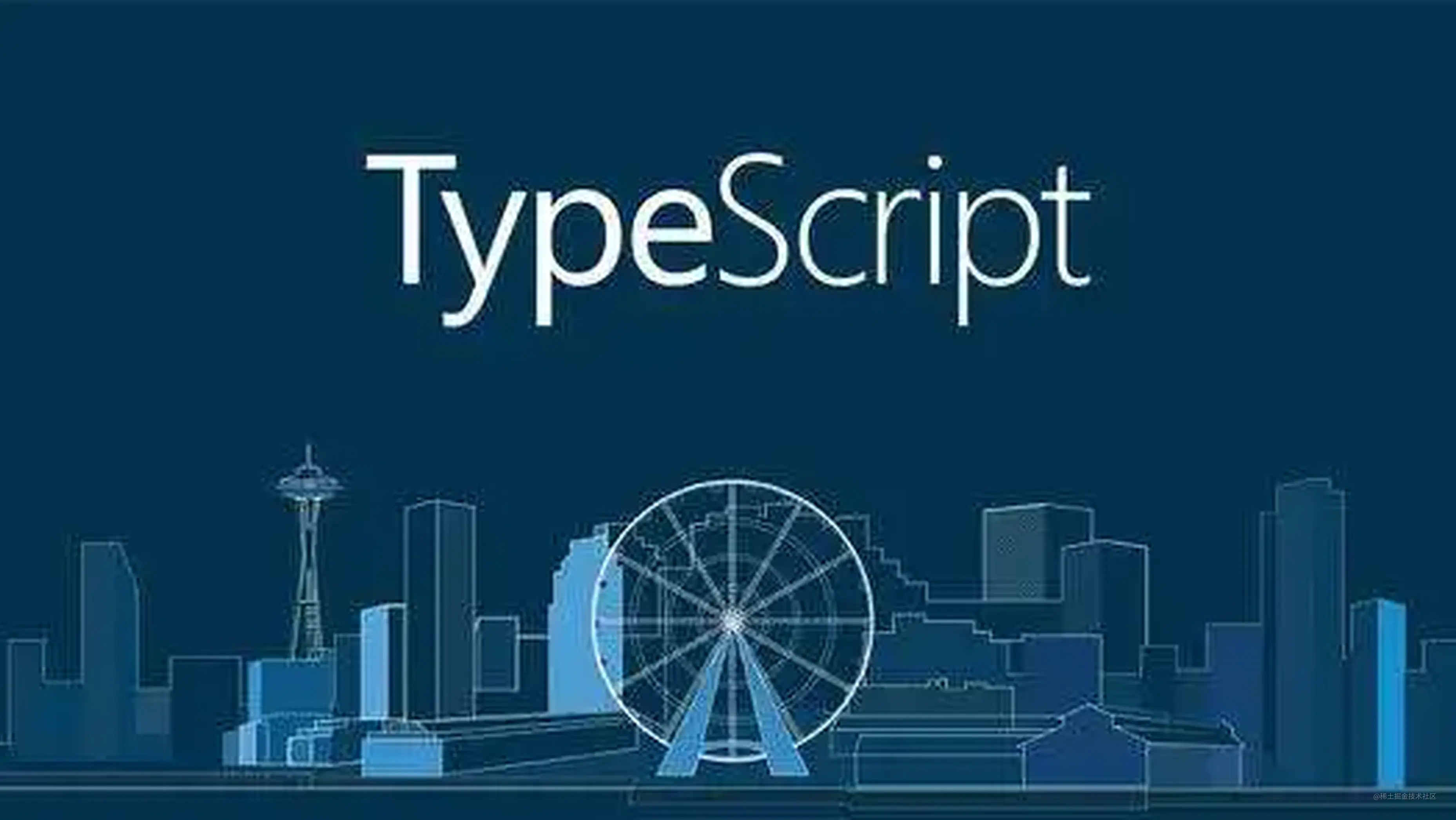# 《二》大话 Typescript 枚举• 数字枚举
• 字符串枚举
• 异构枚举
• 常量枚举
• 枚举成员
• 枚举合并
• 外部枚举
• 总结

#### 数字枚举

``````enum learn {
math,
language,
sports
}
console.log(learn.math) // 0
console.log(learn.language) // 1
console.log(learn.sports) // 2

``````enum learn {
math = 2,
language,
sports
}
console.log(learn.math) // 2
console.log(learn.language) // 3
console.log(learn.sports) // 4

``````var learn;
(function (learn) {
learn[learn["math"] = 2] = "math";
learn[learn["language"] = 3] = "language";
learn[learn["sports"] = 4] = "sports";
})(learn || (learn = {}));

learn["math"] = 2 // 将key值和 value 对应 learn[learn["math"] = 2] = "math" //此时将 value 作为 索引, key 作为 value

#### 字符串枚举

``````enum learn {
Success = '成功!',
Fail= '失败'
}

``````var learn;
(function (learn) {
learn[learn["math"] = 0] = "math";
learn["Success"] = "\u6210\u529F!";
})(learn || (learn = {}));

``````enum learn {
Success = '成功!',
Fail //报错
}

#### 异构枚举

``````enum learn {
math,
Success = '成功!',
}

#### 常量枚举

``````const enum E {
X, Y, Z
}

function f(obj: { X: number }) {
return obj.X;
}

f(E); //报错

``````function f(obj) {
return obj.X;
}
f(E); //报错

``````const enum learn {
math,
language,
sports
}

#### 枚举成员

1. 成员是只读的, 不可以修改
``````enum learn {
math = 2,
language,
sports
}
learn.math = 2  // 报错

1. 枚举成员可以是 常量, 也可以是变量
``````enum learn {
a,
b = 2,
c = 1 + 3, //表达式
e = '123'.length
}

1. 枚举是在运行时真正存在的对象, 可以传递给对象使用
``````enum E {
X, Y, Z
}
function f(obj: { X: number }) {
return obj.X;
}
f(E); //1

#### 最后

• 欢迎加我微信(A18814127),拉你进技术群，长期交流学习
• 欢迎关注「前端加加」,认真学前端，做个有专业的技术人